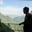Related Tags

tibbles
r

# What are tibbles in R?AKASH BAJWA

### Overview

A fantastic feature that was added to the R language by Hadley Wickham is the modern re-imagination of DataFrames, commonly known as tibbles or tbl_df. Tibbles work like lazy DataFrames. They don't perform a lot of new actions, but purify the older ones by keeping the essential elements and discarding the less effective ones.

Using lazy DataFrames is a way to query data where and when required. It creates a logical plan then return computed data with either the pull(), collect(), or as.data.frame() function.

### Features of tibbles

• They never change the data type.
• They don’t change characters to strings.
• They turn lists to columns.
• They support non-standard variable names, which means they can have a number at the start or even spaces.
• Tibbles only recycle vectors whose lengths are equal to 1.
• They don’t create row names.

### Syntax

This is how we can create a tibble:

# using this method to create tibbles
tibble()
#or
as_tibble()

### Explanation

Let’s elaborate on some coding examples that are associated with the functions given above.

### The tibble() function

library(tibble)
mytibble= tibble(x = 1:4, y = 2, z = x ^ 3 + y)
print(mytibble)
• Line 1: We use the library tibble to perform the required functionality.
• Line 2: We use the tibble() function and pass the vector values to create a DataFrame with four rows and three columns.
• Output: Column x contains values from 1 to 4. Column y has an int value of 2 in all places. Column z applies the formula, calculating x power 3 and then adding the value of y.

#### The as_tibble() function

library(tibble)
mydata <- data.frame(x = 4:8, y = letters[4:8], z = Sys.Date() - 4:8)
print(mydata)
mytibble= as_tibble(mydata)
print(mytibble)
• Line 1: We use the library tibble to perform the required function.
• Line 2: We create a DataFrame under the alias mydata and add values to the three columns and five rows of the DataFrame. Each row has an integer digit starting from 4, an alphabetical letter that corresponds to that number, and the system date after subtracting the respective int value.
• Line 3: We display the DataFrame. This is an optional step in the process.
• Line 4: We convert the DataFrame into a tibble using the as_tibble() function and assign it to the mytibble variable.
• Line 5: We print the tibble to the console.

RELATED TAGS

tibbles
r

CONTRIBUTORAKASH BAJWA
RELATED COURSES

View all Courses

Keep Exploring

Learn in-demand tech skills in half the time